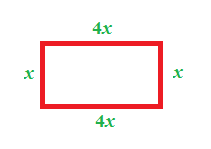### Home > CC2MN > Chapter 6 Unit 6B > Lesson CC2: 6.1.2 > Problem6-18

6-18.

When solving a problem about the perimeter of a rectangle using the 5-D Process, Herman built the expression below.  Homework Help ✎

$\text{Perimeter}=x+x+4x+4x$ feet

1. Draw a rectangle and label its sides based on Herman’s expression.

Use each part of the expression as a side of the rectangle. Remember, rectangles have two pairs of equal, opposite sides. Try creating a rectangle with the given expression.

Figure not drawn to scale.2. What is the relationship between the base and height of Herman’s rectangle? How can you tell?

Examine the rectangle and compare the base and height. Are there any common factors between them that make them related?
If so, how are they different?

3. If the perimeter of the rectangle is 60 feet, how long are the base and height of Herman’s rectangle? Show how you know.

Using the expression Herman created, how can you use the given perimeter to solve for the base and height of the rectangle?

Use the 5-D Process to conduct trial runs with the given expression and perimeter to solve for the unknown variable and as a result, determine the base and height.